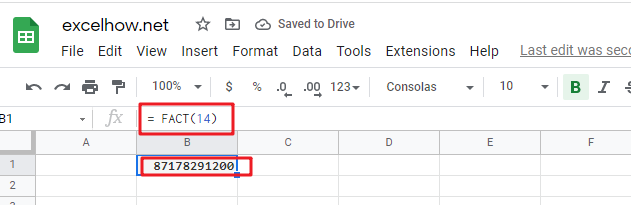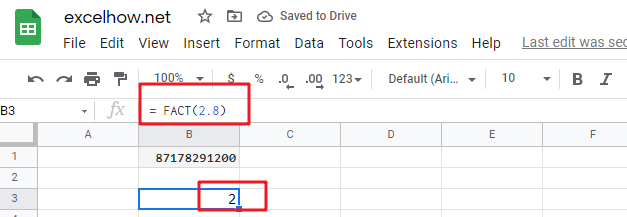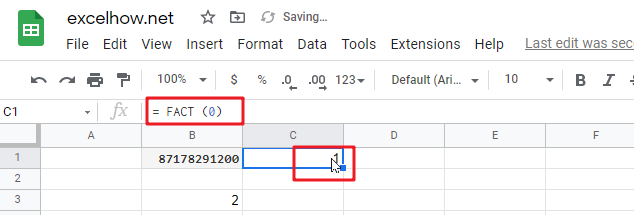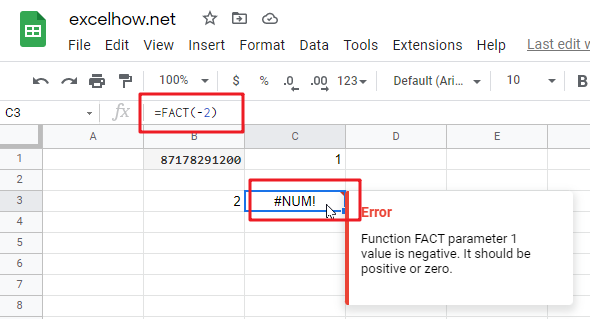This post will guide you how to use Google Sheets FACT function with syntax and examples.

## Description

The Google Sheets FACT function returns the factorial of a given number.  The factorial of a given number is equal to 1*2*3…* number.

The FACT function can be used to find the factorial of a number in google sheets. And its returned value is the factorial of a number.

The FACT function is a build-in function in Google Sheets and it is categorized as a MATH function.

## Syntax

The syntax of the FACT function is as below:

= FACT (number)

Where the FACT function argument is:

• number – This is a required argument. A numeric value that you want to calculate the factorial. And the number need to be a positive integer. If the number is not an integer and it will be truncated.

Note:

• If the number argument is negative, the FACT function will return #NUM! Error.
• If the number argument is non-numeric, the FACT function will return #VALUE! Error.

## Google Sheets FACT Function Examples

The below examples will show you how to use google sheets FACT Function to calculate the natural logarithm of a given number.

1# to get the factorial of number 14, enter the following formula in Cell B1.

`= FACT(14)`2# to get the factorial of the integer of 2.8, enter the following formula in Cell B2.

`= FACT(2.8)`3# to get the factorial of 0, enter the following formula in Cell B3.

`= FACT (0)`4# to get the factorial of a negative number, and returns a #NUM! Error, enter the following formula in Cell B4

`=FACT(-2)`